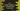# C++ program to find the nearest smaller number to the left side in an array## C++ program to find the nearest smaller number to the left side in an array:

This post will show you how to find the nearest smaller number to the left side in an array using C++. The program will take one array of integers as the input and it will print another array holding the nearest smaller on left side for each element.

For example, if the array is:

``[1, 4, 0, 5, 15, 6]``

It will print:

``[-1, 1, -1, 0, 5, 5]``

For 1, we don’t have any number to the left which is smaller that it. So it adds -1. For 4, we have 1. For 0, we don’t have. For 5, we have 0, for 15 we have 5 and for 6, we have 5.

We will learn two different ways to solve this problem. One by using two for loops and another by using a stack.

### By using two for loops:

``````#include <iostream>
using namespace std;

void findAllSmaller(int givenArr[], int size, int finalArray[])
{
finalArray = -1;

for (int i = 1; i < size; i++)
{
finalArray[i] = -1;
for (int j = i - 1; j >= 0; j--)
{
if (givenArr[j] < givenArr[i])
{
finalArray[i] = givenArr[j];
break;
}
}
}
return;
}

int main()
{
int givenArray[] = {1, 4, 0, 5, 15, 6};
int size = sizeof(givenArray) / sizeof(givenArray);

int finalArray[size];

findAllSmaller(givenArray, size, finalArray);

for (int i = 0; i < size; i++)
{
cout << finalArray[i] << " ";
}
return 0;
}``````

Here,

• findAllSmaller method is used to find all smaller numbers to the left side of each number in the array.
• This method takes the original array, size and the new array as arguments. It fills the required values in the new array.
• Inside findAllSmaller, we are using two loops. The inner loop finds the smaller element and fills these in finalArray. It assigns the current element value as -1. Then it iterates to left and if any smaller value is found, it updates the array.
• Once findAllSmaller is done, we can print the contents of finalArray, which is the required array.

If you run the above program, it will print the below output:

``-1 1 -1 0 5 5``

It’s complexity is O(n^2).

## By using a stack:

We can also solve this by using a stack. This will solve this problem in O(n) complexity.

Below is the algorithm we need to use:

1. Create one empty stack
2. Iterate through the array elements one by one. For each element,

1. Keep popping the stack, while the stack is non-empty and the top element is greater than or equal to the current element.
2. If the stack is empty, current element has no smaller value to its left. Else, the top element is the nearest preceding smaller value for the current element.
3. Push the current element to the stack.

For stack, we can use #include header file in C++.

### C++ program:

Below is the complete program:

``````#include <iostream>
#include <stack>
using namespace std;

void findAllSmaller(int givenArr[], int size, int finalArray[])
{
int currentIndex = 0;
stack<int> stack;

for (int i = 0; i < size; i++)
{
while (!stack.empty() && stack.top() >= givenArr[i])
stack.pop();

if (stack.empty())
finalArray[currentIndex] = -1;
else
finalArray[currentIndex] = stack.top();

stack.push(givenArr[i]);
currentIndex++;
}
return;
}

int main()
{
int givenArray[] = {1, 4, 0, 5, 15, 6};
int size = sizeof(givenArray) / sizeof(givenArray);

int finalArray[size];

findAllSmaller(givenArray, size, finalArray);
for (int i = 0; i < size; i++)
{
cout << finalArray[i] << " ";
}
return 0;
}``````

Here,

• We are using the same method name as the previous example.
• currentIndex variable is used to keep the current index of the finalArray. It is initialized as 0.
• The while loop is used to keep popping the stack while it iis not empty and the top element is greater than or equal to the current element.
• The if-else block checks if the stack is empty or not and based on that it updates the finalArray.
• The current element is pushed to the stack and currentIndex is incremented by 1 on each iteration of the for loop.

If you run this program, it will print similar output.